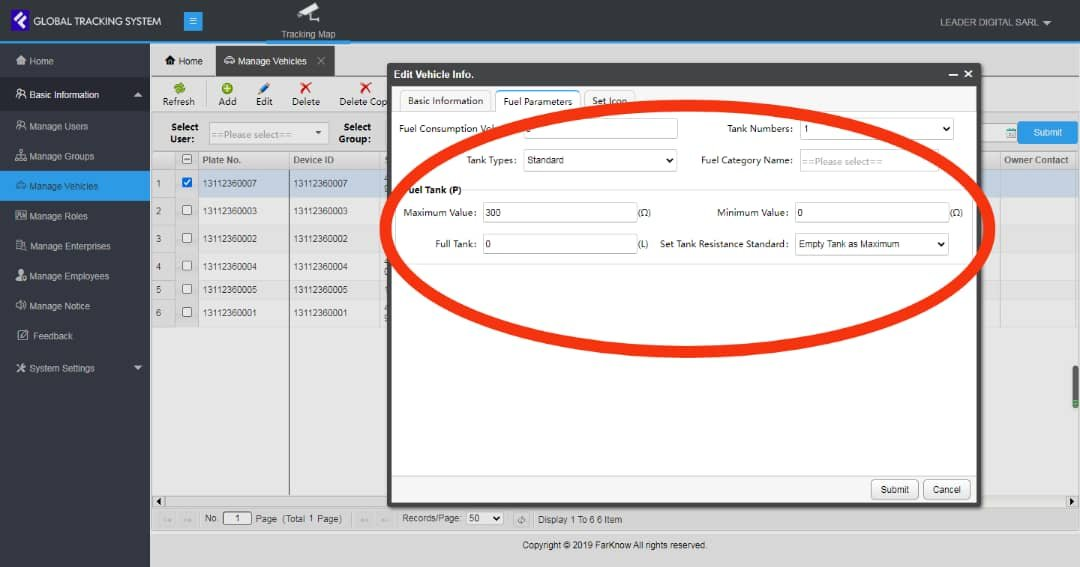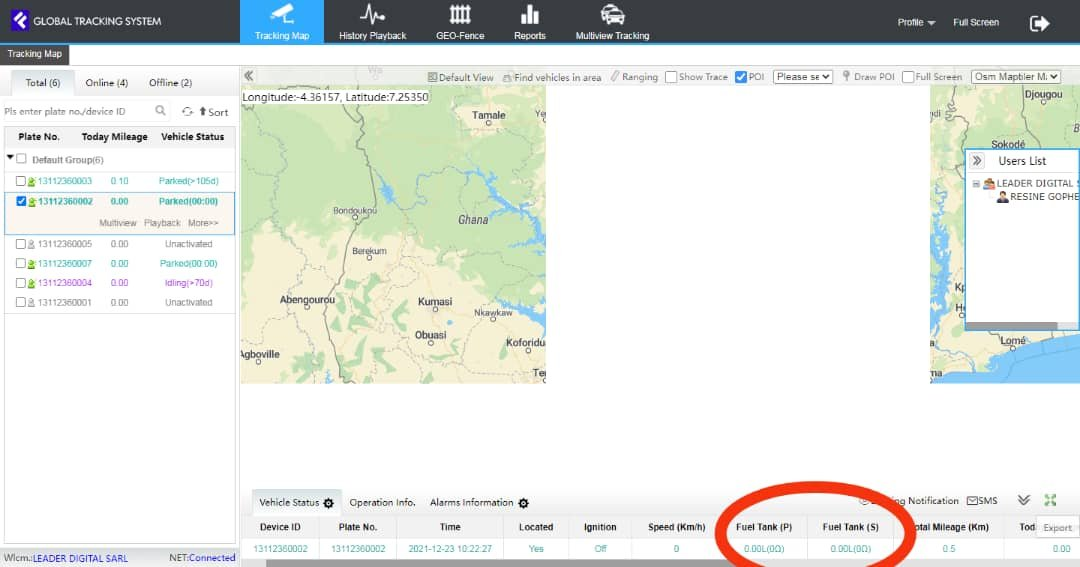# How to configure the fuel sensor?

Explanation:

The first step is to configure the parameters in the platform2. We know the full tank fuel volume from trucks official information. We call it V3

3. Never mind how much fuel is left in tank, we could know how much volume we add fuel to full
tank, we call the volume amount V2, we can get to know from fuel station.

4. Before we add fuel to full tank, we can read the resistance of the fuel that left in tank, we call it
R1.5. When add fuel to full tank, we can read the resistance of full tank fuel, we call it R3. This R3 is
the maximum resistance if the tank’s resistance is as per the trend of increasing with fuel.

6. The Resistance of the fuel you just added to full, we call it R2, R2=R3‐R1.

7. We now don’t know the minimum resistance, we call it X and it’s the value we need to
calculate out.

8. the formula is like below:

R3‐X/V3=R2/V2

R3 (Read out value in platform)

V3 (Get to know from official information)

R2(Calculated by R3 and R1, R1 read out from platform)

V2 (Get to know from fuel station)

So X=R3‐V3*R2/V2=R3‐V3*(R3‐R1)/V2

When we calculate out X, the minimum resistance, then we can put into the parameters, the fuel
chart will be correct.